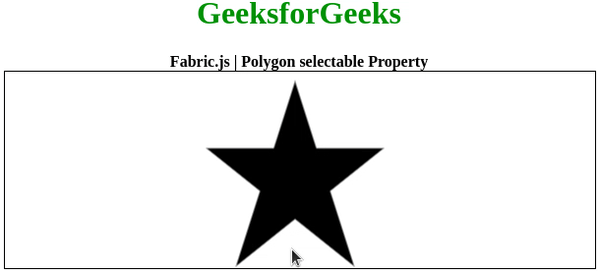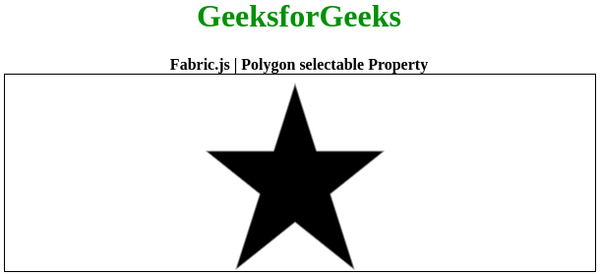# Fabric.js Polygon selectable Property

• Last Updated : 09 Feb, 2021

In this article, we are going to see how to set the selectability of a canvas polygon using FabricJS. The canvas polygon means the polygon is movable and can be stretched according to requirement. Further, the polygon can be customized when it comes to initial stroke color, shape, fill color, or stroke width.

To make it possible we are going to use a JavaScript library called FabricJS. After importing the library, we will create a canvas block in the body tag that will contain the polygon. After this, we will initialize instances of Canvas and polygon provided by FabricJS and set the selectability of the polygon using selectable property and render the polygon on the Canvas as given in the example below.

Syntax:

```fabric.Polygon([
{ x: pixel, y: pixel },
{ x: pixel, y: pixel },
{ x: pixel, y: pixel },
{ x: pixel, y: pixel },
{ x: pixel, y: pixel }],
{
selectable: boolean
}
);```

Parameters: This property accepts a single parameter as mentioned above and described below:

• selectable: It specifies whether to selectable the canvas or not.

Note: Dimension pixels is must to create a polygon.

Below examples illustrate the Fabric.JS Polygon selectable property in JavaScript:

Example 1: Here the selectable property is disabled.

## HTML

 ```<``html``>``<``head``>``    ````    ``<``script` `src``=``"https://cdnjs.cloudflare.com/ajax/libs/fabric.js/3.6.2/fabric.min.js"``>``    ````` `<``body``>``    ``<``div` `style``=``"text-align: center;width: 600px;"``>``        ``<``h1` `style``=``"color: green;"``>``            ``GeeksforGeeks``        ````        ``<``b``>``            ``Fabric.js | Polygon selectable Property``        ````    ``` `    ``<``canvas` `id``=``"canvas"``            ``width``=``"600"``            ``height``=``"200"``            ``style``=``"border:1px solid #000000;"``>``    ``` `    ``<``script``>` `        ``// Initiate a Canvas instance``        ``var canvas = new fabric.Canvas("canvas");` `        ``// Initiate a polygon instance``        ``var polygon = new fabric.Polygon([``        ``{ x: 295, y: 10 },``        ``{ x: 235, y: 198 },``        ``{ x: 385, y: 78},``        ``{ x: 205, y: 78},``        ``{ x: 355, y: 198 }], {``            ``selectable: false``        ``});` `        ``// Render the polygon in canvas``        ``canvas.add(polygon);``    ````` ``

Output:Example 2: Here the selectable property is enabled.

## HTML

 ```<``html``>``<``head``>``    ````    ``<``script` `src``=``"https://cdnjs.cloudflare.com/ajax/libs/fabric.js/3.6.2/fabric.min.js"``>``    ````` `<``body``>``    ``<``div` `style``=``"text-align: center;width: 600px;"``>``        ``<``h1` `style``=``"color: green;"``>``            ``GeeksforGeeks``        ````        ``<``b``>``            ``Fabric.js | Polygon selectable Property``        ````    ``` `    ``<``canvas` `id``=``"canvas"``            ``width``=``"600"``            ``height``=``"200"``            ``style``=``"border:1px solid #000000;"``>``    ``` `    ``<``script``>` `        ``// Initiate a Canvas instance``        ``var canvas = new fabric.Canvas("canvas");` `        ``// Initiate a polygon instance``        ``var polygon = new fabric.Polygon([``        ``{ x: 295, y: 10 },``        ``{ x: 235, y: 198 },``        ``{ x: 385, y: 78},``        ``{ x: 205, y: 78},``        ``{ x: 355, y: 198 }], {``            ``selectable: true``        ``});` `        ``// Render the polygon in canvas``        ``canvas.add(polygon);``    ````` ``

Output:My Personal Notes arrow_drop_up